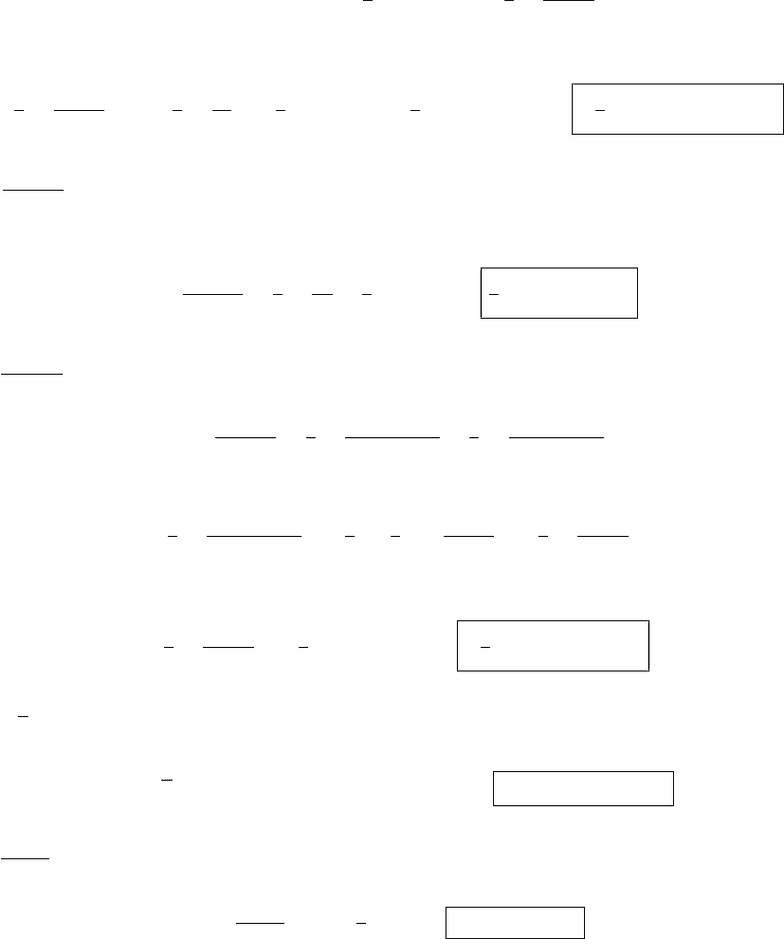# MTH 151 Study Guide - Midterm Guide: Tasmanian Government Railways Y Class, Scilab, Hyperbolic Function

49 views6 pages
School
Department
Course
ProfessorName:
Exam 3 - Math 151
Dec 14/15, 2009
R. Ketchersid
Instruction. I would like you to perform your scratch work on scratch paper, I want to see neat work on
the exams, however, all relevant work must be shown unless I believe the problem is so simple that it does
not require any work. When you do a substitution you should write out what you are doing carefully so that
I can follow your line of reasoning.
Put a box around your ﬁnal answer so it is clear to me what you want me to grade. If your answer is hard
to ﬁnd or ambiguous, then you will loose points.
You may skip the ﬁnal step in the evaluation of a deﬁnite integral. So if your problem is to evaluate
R3
2tt21dt, then I expect something like the following for a deﬁnite integral:
Let u=t21so du/2 = t dt and
Z3
2
tpt21dt =1
2Z8
3
u du = (1/2)(2/3)u3/2
8
3
and something like the following for an indeﬁnite integral:
Let u=t21so du/2 = t dt and
Ztpt21dt =1
2Zu du = (1/2)(2/3)u3/2+C= 1/3(t21)3/2+C
If you have questions on the form of your answer please ask during class. As a rule of thumb, there is no
question on this exam that requires a calculator; in fact I expect exact answers to questions not numeric
approximations.
d
dx cosh1(u) = 1
u21
du
dx (u > 1) d
dx sinh1(u) = 1
u2+ 1
du
dx
d
dx coth1(u) = 1
1u2
du
dx (|u|>1) d
dx tanh1(u) = 1
1u2
du
dx (|u|<1)
d
dx csch1(u) = 1
|u|1 + u2
du
dx (u6= 0) d
dx sech1(u) = 1
u1u2
du
dx (0 < u < 1)
Unlock document

This preview shows pages 1-2 of the document.
Unlock all 6 pages and 3 million more documents.

Already have an account? Log in-2-
1)[42pts, 3pts each] Evaluate the following deﬁnite and indeﬁnite integrals.
a) Zt2tan(3t3+ 2) dt
Let u= 3t3+ 2 so du/dt = 9t2hence 1/9du =t2dt and
Zt2tan(3t3+ 2) dt =1
9Ztan(u)du =1
9Zsin(u)
cos(u)du
Let v= cos(u)so dv =sin(u)du and
1
9Zsin(u)
cos(u)du =1
9Zdv
v=1
9ln |v|+C=1
9ln |cos(u)|+C=1
9ln |cos(3t3+ 2)|+C
b) Zy dy
4y29
Let u= 4y29so du/dy = 8yhence 1/8du =y dy and
Zy dy
4y29=1
8Zdu
u=1
8ln |u|+C=1
8ln |4y29|+C
c) Zdu
4u29with 4u29>0
Zdu
4u29=1
9Zdu
(4/9)u21=1
9Zdu
(2/3u)21
Let v= 2/3uso 3/2dv =du and
1
9Zdu
(2/3u)21=1
93
2Zdv
v21=1
6Zdv
1v2
Notice 4u29>0is equivalent to |v|>1so we have
1
6Zdv
1v2=1
6coth1(v) + C=1
6coth1(2/3u) + C
d) Z3
u(2u1/u)du
Z3
u(2u1/u)du =Z2u4/3u2/3du = 6/7u7/33u1/3+C
e) Z23t
tdt
Z23t
tdt =Z2
t3dt = 2 ln |t| − 3t+C
Unlock document

This preview shows pages 1-2 of the document.
Unlock all 6 pages and 3 million more documents.

Already have an account? Log in

# Get OneClass Grade+

Unlimited access to all notes and study guides.

###### Grade+All Inclusive
\$10 USD/m
You will be charged \$120 USD upfront and auto renewed at the end of each cycle. You may cancel anytime under Payment Settings. For more information, see our Terms and Privacy.
Payments are encrypted using 256-bit SSL. Powered by Stripe.